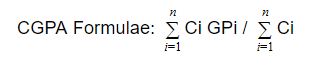## How to Calculate CGPA to Percentage?How to Calculate CGPA to Percentage: The full form of CGPA is Cumulative Grade Point Average. The University Grants Commission (UGC), an official government body has set some pre-designed measures to introduced efficient grading in the higher education system of India. CGPA provides average grade points for the performance of the student. It has a ratio quantity which is expressed up to two decimal places.

CGPA is one such measure used vividly for the grading system in central, state universities in India for undergraduate and postgraduate degrees, diplomas and other certification programs. Many schools and boards use a grading system to evaluate an individual’s academic performance. This score of yours is the first and foremost factor every admissions committee considers in determining eligibility to apply to colleges.

## What is CGPA?

CGPA is an abbreviation for Cumulative Grade Point Average. It is a grading system used in the educational field. It is generally used to assess a student’s overall academic achievement in a particular subject. The cumulative grade point average (CGPA) is calculated by taking the average of the GPA a student receives each semester and dividing it by the total number of credits.

## Difference between CGPA and GPA

GPA measures performance in a single unit or semester, and CGPA measures performance across a course, including all units and semesters. In this article we will share with you “How to Calculate CGPA to Percentage”.

## How to Calculate CGPA?

Your CGPA is calculated by dividing the sum of the grade points earned by the total credit value of course you have attempted.## How to Calculate CGPA to Percentage?

In order to convert cgpa to percentage, follow these steps below –

• Next, divide the total grades by the total number of subjects.
• You will get the CGPA.
• Finally, multiply the CGPA by 9.5 to get the percentage equivalent.
91-100 A1 10.0
81-90 A2 9.0
71-80 B1 8.0
61-70 B2 7.0
51-60 C1 6.0
41-50 C2 5.0
33-40 D 4.0
21-32 E1
00-20 E2

## How to Convert CGPA into Marks?

To convert cpga into marks you need to follow these steps –

• First convert the CGPA into percentage.
• Next, multiply the obtained CGPA by 9.5.
• You will get the percentage.
• Now multiply the percentage/100 with total maximum marks of all subjects.

Example:- Suppose the CGPA=6.4 and the overall percentage (%) obtained = 6.4*9.5=60.8%. So, if the percentage is 60.8%, then total marks obtained 60.8/100*500= 304.

## How to Calculate Percentage to CGPA?

As per the Indian grading system, the conversion of Percentage (%) to CGPA varies slightly from one university to another and differs from one institution to another. Following is the general formula for calculating Cumulative Grade Point Average (CGPA) in Engineering or any other course in India: Percentage/9.5 = CGPA